# Contemporary Mathematics

Mathematics

## Quiz 13 : Consumer and Business CreditStudy FlashcardsLooking for Developmental Mathematics Homework Help?

## Quiz 13 :Consumer and Business Credit

Question TypeLoans made on a continuous basis and billed periodically are known as _____ credit.
Free
Essay

Revolving credit:
Loan made on a continuous basis and billed periodically. Borrower makes minimum monthly payments or more and pays interest on the outstanding balance. This is a form of open-end credit extended by many retail stores and credit card companies.

Tags
Choose question tagChris Manning purchased a $7,590 motorcycle with a 36-month installment loan. The monthly payments are$261.44 per month. a. Use the APR formula to calculate the annual percentage rate of the loan. Round to the nearest hundredth of a percent. b. Use the APR tables to verify your answer from part a.
Free
Essay

The given data is the amount financed is $7,590.00 The number of payments is 36 And monthly payment is$261.44
To find the finance charge:
The finance charge can be found by subtracting the amount financed from the total amount of installment payments
That means,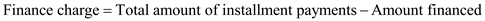To calculate the finance charge, we must find the total amount of installment payments
To find the total amount of installment payments:
When the amount of the monthly payments is known, the total amount of installment payments can be found by multiplying the monthly payment amount by the number of payments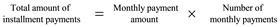Since, by given information, the monthly payment amount is $261.44 And the number of payments is 36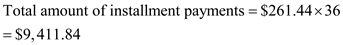For finance charge,So, the finance charge is$1,821.84
a) To find annual percentage rate:
When annual percentage rate (APR) tables are not available, the annual percentage rate can be closely approximated by the formula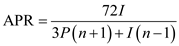Here, I is the finance charge on the loan
P is the principal, or amount financed
And n is the number of months of the loan
By given hypothesis,By substituting these values in the APR formula,So, the annual percentage rate of the loan is 14.47%
b) To verify the answer by using APR table:
Calculate the finance charge per $100.So, the finance charge per$100 is $24.00 Using table 13-1, scan down the number of payments column to 36 payments Scan to the right in that number of payments row until you find$24.00, the finance charge per $100 Looking at the top of the column containing the$24.00, you will find the annual percentage rate for the loan to be 14.75%
Therefore, the annual percentage rate of the loan is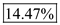And the annual percentage rate by using table is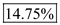Tags
Choose question tagCalculate the average daily balance for January of a charge account with a previous month's balance of $480.94 and the following activity.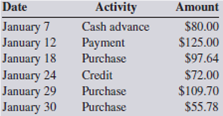Free Essay Answer: Answer: To calculate the daily balances and their sum, set up a chart like the one below that lists the activity in the account by dates and number of days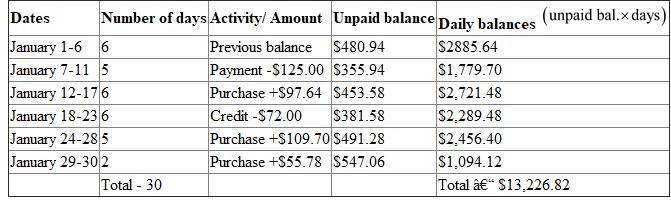Now to find the average daily balance: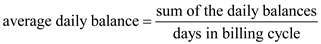Here, the sum of the daily balances is$13,226.82
And days in billing cycle is 30Therefore, the average daily balance for the month of January is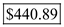.
To find the periodic rate:
Divide the annual percentage rate by 12 to find the monthly or periodic interest rate.So, the annual percentage rate is 1.08%
b) To find financial charge:
Calculate the finance charge by multiplying the previous month's balance by the periodic interest rate from step 1.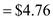Therefore, the finance charge is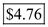c) To find the new balance:
Total all the purchases and cash advances for the month
All the purchases and cash advances for the month is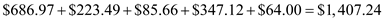Total all the payments and credits for the month
Since, given that all the payments and credits for the months is $550 Use the following formula to determine the new balance:Therefore, the average daily balance isThe new balance is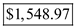The finance charge of charge in the month of September is. Tags Choose question tagGeorge Bell bought an ultralight airplane for$29,200. He made a 15% down payment and financed the balance with payments of $579 per month for 60 months. a. What is the finance charge on George's loan? b. What is the total deferred payment price of the airplane? Essay Answer: Tags Choose question tagWrite the formula for calculating the average daily balance of a revolving credit account. Essay Answer: Tags Choose question tagDaniel Noguera has a Bank of America revolving credit account with an annual percentage rate of 12% calculated on the previous month's balance. In April, the account had the following activity.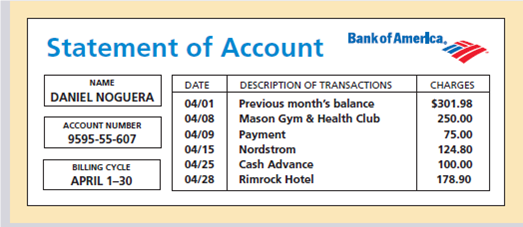a. What is the finance charge? b. What is Daniel's new balance? Essay Answer: Tags Choose question tagThe interest rate of most lines of credit is tied to the movement of the _____ rate. Essay Answer: Tags Choose question tagThe portion of the purchase price of an asset paid in a lump sum at the time of purchase is known as the _____ payment. Essay Answer: Tags Choose question tagA loan made for a specified number of equal monthly payments is known as a(n) _____ loan. Essay Answer: Tags Choose question tagSound Blaster Recording Studio purchased a new digital recording console for$28,600. A down payment of $5,000 was made and the balance financed with monthly payments of$708 for 48 months. a. What is the finance charge on the loan? b. Use Table 13-1 to find what annual percentage rate was charged on the equipment loan.
Essay
Tags
Choose question tag_____ credit is a loan arrangement in which there is no set number of payments.
Essay
Tags
Choose question tagDavid Sporn bought a saddle from Linville Western Gear with a 9.3% addon interest installment loan. The purchase price of the saddle was $1,290. The loan required a 15% down payment and equal monthly payments for 24 months. a. What is the total deferred payment price of the saddle? b. What are David's monthly payments? Essay Answer: Tags Choose question tagThe effective or true annual interest rate being charged for credit is known as the _____ _____ _____ and is abbreviated _____. Essay Answer: Tags Choose question tagMel Arrandt has a Bank of America account with a 13% annual percentage rate calculated on the average daily balance. The billing date is the first day of each month, and the billing cycle is the number of days in that month.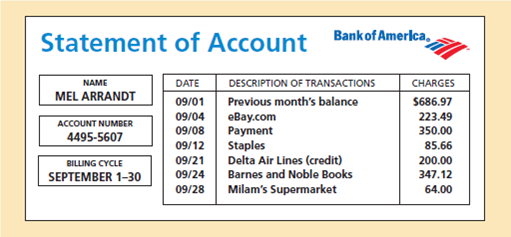a. What is the average daily balance for September? b. What is the finance charge for September? c. What is Mel's new balance? Essay Answer: Tags Choose question tagHeather MacMaster's revolving credit account has an annual percentage rate of 16%. The previous month's balance was$345.40. During the current month, Heather's purchases and cash advances amounted to $215.39 and her payments and credits totaled$125.00. a. What is the monthly periodic rate of the account? b. What is the finance charge? c. What is Heather's new balance?
Essay
Tags
Choose question tagName the two most common methods used to calculate the finance charge of a revolving credit account.
Essay
Tags
Choose question tagLoans that are backed by the borrower's "promise" to repay are known as _____ loans, whereas loans that are backed by a tangible asset are known as _____ loans.
Essay
Tags
Choose question tagA pre-approved amount of open-end credit is known as a(n) _____ of credit.
Essay
Tags
Choose question tagCharlotte Williams has a Visa account. The finance charge is calculated on the previous month's balance, and the annual percentage rate is 20%. Complete the following three-month account activity table for Charlotte.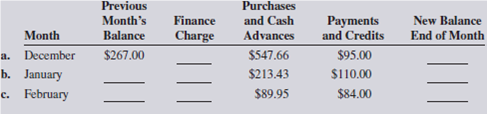EssayAlpine Construction, Inc., has a $100,000 line of credit with the Bow Valley Bank. The annual percentage rate is the current prime rate plus 31 4%. The balance on June 1 was$52,900. On June 8, Alpine borrowed $30,600 to pay for a shipment of lumber and roofing materials and on June 18 borrowed another$12,300 for equipment repairs. On June 28, a \$35,000 payment was made on the account. The billing cycle for June has 30 days. The current prime rate is 73 4%. a. What is the finance charge on the account? b. What is Alpine's new balance?